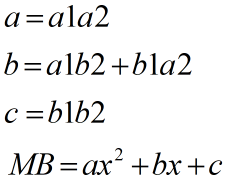Request a Tool

Multiply Binomials Calculator

Multiply Binomials Calculator is use to calculate binomial equation from a1, b1, a2 and b2.

Equation
0

Formula• MB = Multibinomial
• a1 = Values of first a
• b1 = Values of first b
• a2 = Values of second a
• b2 = Values of second b

Defination / Uses

A multinomial experiment is nearly identical to a binomial experiment with one major difference: a binomial experiment can only have two results, whereas a multinomial experiment can have many. Example: To find out what number you roll, you roll a die ten times. This is a multinomial experiment because there are six choices (1, 2, 3, 4, 5, 6).

Welcome to the multiplying binomials calculator, where we'll learn how to multiple binomials, as the name implies. Although it is a subset of polynomial multiplication, it is so widely used in applications and textbooks that it needed its own calculator. So, for the time being, binomials are the only polynomials we need (kind of...), and just in case you're not sure what they are, we've included the binomial definition in math. Mathematics is known as the "universal language" because it describes the laws that govern the cosmos. Sure, physicists do the same thing, but at the end of the day, physics is merely math applied to specific conditions.